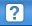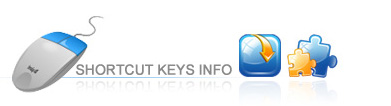Shortcut Key InfoWelcome to IconCool Studio Shortcut Key Info
In IconCool Studio, quicker and easier shortcut keys processing offer you the same impressed image effect and save your precious time.
All the shortcut keys and functions are listed specifically as follows:
• Ctrl + N =New
• Ctrl +O =Open
• Ctrl +S =Save
• Ctrl +W =Close
• Ctrl +Q =Exit
• Ctrl + Z =Undo
• Ctrl +Y =Redo
• Ctrl +X =Cut
• Ctrl +C =Copy
• Ctrl +V =Paste
• Del =Delete
• Ctrl +A =Select All
• Ctrl +D =Deselect
• F4 =Resize the Image
• F3 =Create New Project...
• Ctrl +Del =Remove the Format
• F5 =Icon Properties
• N = Invert
• V =Move Tool
• L =Line
• P =Pencil
• B =Brush
• R =Rectangle
• F =Filled Rectangle
• X=Ellipse
• D =Filled Ellipse
• C =Color Picker
• A = Paint Bucket
• E =Eraser Tools
• S =3D Frame
• G =Lighting Effect